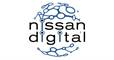Stack and Queue <Nissan>
Tag(s):

## Arrays, Data Structures, Easy, Implementation, Queue, Stack, Stack

Problem
Editorial
Analytics

You are given a stack of N integers such that the first element represents the top of the stack and the last element represents the bottom of the stack. You need to pop at least one element from the stack. At any one moment, you can convert stack into a queue. The bottom of the stack represents the front of the queue. You cannot convert the queue back into a stack. Your task is to remove exactly K elements such that the sum of the K removed elements is maximised.

Input format :

• The first line consists of two space-separated integers N and K.
• The second line consists of N space-separated integers denoting the elements of the stack.

Output format :

• Print the maximum possible sum of the K removed elements

Constraints :

• $1 \le N \le 10^{5}$
• $1 \le K \le N$
• $1 \le A_{i} \le 10^{9}$
SAMPLE INPUT
10 5
10 9 1 2 3 4 5 6 7 8
SAMPLE OUTPUT
40
Explanation

Pop two elements from the stack. i.e {10,9}

Then convert the stack into queue and remove first three elements from the queue. i.e {8,7,6}.

The maximum possible sum is 10+9+8+7+6 = 40

Time Limit: 1.0 sec(s) for each input file.
Memory Limit: 256 MB
Source Limit: 1024 KB
Marking Scheme: Marks are awarded when all the testcases pass.
Allowed Languages: Bash, C, C++, C++14, Clojure, C#, D, Erlang, F#, Go, Groovy, Haskell, Java, Java 8, JavaScript(Rhino), JavaScript(Node.js), Julia, Kotlin, Lisp, Lisp (SBCL), Lua, Objective-C, OCaml, Octave, Pascal, Perl, PHP, Python, Python 3, R(RScript), Racket, Ruby, Rust, Scala, Swift, Swift-4.1, TypeScript, Visual Basic

## CODE EDITOR

Initializing Code Editor...

## This Problem was Asked inChallenge Name

Nissan Digital Software Developer Hiring Challenge

OTHER PROBLEMS OF THIS CHALLENGE
• Algorithms > Searching
• Basic Programming > Bit Manipulation